telescopeѲptics.net          ▪▪▪▪                                             CONTENTS

# 6.7. COHERENT TRANSFER FUNCTION, FOURIER TRANSFORM

As mentioned before, optical transfer function (OTF) is defined for incoherent light, hence so are its elements, including MTF. While the light entering telescopes is generally incoherent, it can not be considered fully incoherent, and the degree of its incoherence varies (spatial coherence, according to Van Cittert-Zernike Theorem, can vary with the apparent object size). As mentioned in 2.2. Telescope resolution, there is no difference in the relative intensity distribution between incoherent and coherent light in imaging a point source, as long as it is near-monochromatic in incoherent light, but there are significant differences in imaging multiple close point sources and extended objects. In that context, it may be interesting to see what effect on contrast transfer would have change of illumination from incoherent to coherent.

In comparing transfer function for coherent and incoherent light, the difficulty arises from different natures of the two. While image of an object in incoherent light forms by superimposing point intensity distribution (system PSF) over Gaussian image of the object, with the intensities at each point directly adding up, with coherent light the image forms by superimposing point image amplitude distribution (coherent spread function, CSF) on every point of Gaussian image, with complex amplitudes at each point adding up to the final point complex amplitude (in that context, we are talking about amplitude image). Squaring this complex amplitude image produces intensity distribution over object's image.

In other words, image in incoherent light is the convolution of the Gaussian (intensity) image and the system PSF, while image in coherent light is the convolution of the Gaussian (amplitude) image and the system CSF. While in the former intensity forms as a superposition of individual waves, which randomly varying phase relationship, in the latter individual waves have constant phase relationship, and interfere building up complex amplitude, the square of which is intensity (Inset G).

Due to its different mechanism of image formation, the standard (incoherent) OTF formalism cannot be applied to coherent light The standard transfer function for coherent light - coherent transfer function (here abbreviated by ATF - for amplitude transfer function - to make it different from the Contrast Transfer Function in the abbreviation) - describes the transfer of amplitude, not intensity. More importantly, coherent light diffracts differently with different object intensity profiles, making ATF much more limited to the special case of a sinusoidal object amplitude distribution, than OTF. For instance, if sinusoidal pattern is replaced by square wave pattern (sharply outlined black and white bars), coherent light diffracts differently and is likely to produce blurred edges (Gibbs effect, or ringing edges).

Lastly, ATF neglects phase disturbances that arise from uneven object surface, producing random interference pattern of bright and dark spots - speckle structure - which can significantly worsen image definition.

As such, ATF is much less useful as a general indicator of image quality than the OTF. For the same sinusoidal OTF-like pattern - which is this time the amplitude, not intensity - ATF gives a very different transfer profile. Optical context from which ATF is derived is illustrated below. Since its key component is Fourier transform of the pupil function, it is followed with a brief outline of the concept of Fourier transforms.

For aberration-free aperture, there is no drop in the amplitude transfer for the entire range of frequencies up to the cutoff frequency. However, the cutoff frequency is 1/2λF, only half the resolution power in incoherent light.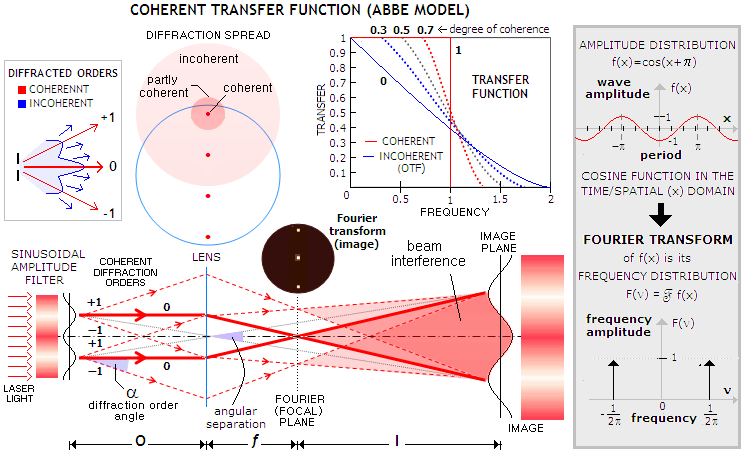This doesn't seem logical, since it appears that diffraction vanishes up to the cutoff frequency, only to entirely block amplitude transfer in an instant, at the twice lower frequency. In fact, there is a lot of diffracting taking place, as illustrated at left, but in this particular case diffraction is sort of "undoing" itself - at list up to a point. At the sinusoidal amplitude filter, coherent light diffracts in a peculiar way, with only two diffraction orders splitting some energy away from the zero order. The angle of diffraction is given by sinα=λ/w, with λ being the wavelength of light and w the line width. As long as the two side diffraction orders pass through the pupil, they will come together forming beam interference with each other and the zero order beam at the image plane, forming sinusoidal intensity distribution, modified vs. that of the object only by the system magnification. As the grating frequency increases, the diffraction orders spread wider; they just miss the pupil at the frequency 1/2λF, and the transfer function abruptly falls to zero.

For the resolved image to form it is sufficient that only a single diffraction order passes through, and combines with the zero-order at the image plane. This means that the cutoff frequency can be up to twice as high - equal to that for incoherent light (1/λF) - if amplitude filter is tilted with respect to the lens.

Unlike coherent, diffracted incoherent light fills the entire pupil, so that a portion of it still passes through the pupil as the diffraction spread widens (top left), up to a frequency twice higher than for coherent light. However, as the signal passing through becomes more and more incomplete, its contrast transfer is steadily declining toward the high frequency cutoff, which is double that for the ATF, since less and less of information from the object reaches the image. For light that is neither coherent or incoherent - taking various degrees of partly coherent, or partly incoherent - the transfer function falls somewhere in between (top right). It should be noted that partly coherent/incoherent transfer functions are, similarly to ATF for coherent light - and unlike OTF - quite limited as indicators of image quality. and cannot be considered valid for a wide range of object/image forms.

In the above illustration, the grating is located at the system aperture, and it is where the diffraction is taking place - not at the lens. Note that the size of the grating is greatly exaggerated for clarity; close to the ATF transfer limit, the two adjoining maximas would be much closer together, and the two triangles formed by their respective +1/-1 diffraction orders would be nearly coinciding, with their points of origin located close to the central axis. Hence the geometry determining cutoff frequency wc comes from sinα=λ/wc=d/c, where d is the aperture radius and c is the triangle hypotenuses. That yields wc=cλ/d and, since the linear value of wc changes with grating distance, with its angular value remaining unchanged, we can chose a convenient value for c to express wc. Such value is c=f (f being the lens focal length), for which the infinitely large image forms at infinity, with wc=2λF and its angular value - as the angle whose base is wc and the tip is center of the objective (in the thin lens concept) is given by wc/f i.e. 2λ/D radians - twice lower cutoff frequency than for incoherent light.

This is also the setup for which the pattern formed in the focal (Fourier) plane is exactly the Fourier transform of the sinusoidal pattern. The lens is often referred to as Fourier transform lens, but it is diffraction at the amplitude filter (grating) that does the transform; the lens merely brings the transform pattern from infinity (or, approximately, Fraunhofer domain) to its focal plane. The 3-point intensity pattern illustrated in the focal plane of the lens is proportional to the function representing the frequency distribution of the cosine function shown in the shaded inset at right. Since the cosine function consists of a single frequency, its frequency amplitude spectrum  (i.e. Fourier transform) is graphically represented as a single delta function (vertical bar) at the unit frequency - called fundamental frequency, as the reciprocal of the period of the cosine function - and unit (in relative terms) frequency amplitude. Expanding frequency spectrum into negative domain is not necessary to define the Fourier transform, but it is a common practice, since it simplifies related expressions and calculation.

In general, frequency amplitude distribution F(ν) - commonly referred to as frequency spectrum - of the fundamental frequency and harmonics that reproduce (or, in the opposite direction, decompose) a function f(x) in the time/spatial domain (i.e. with the variable changing as a function of time, and/or space) is its Fourier transform, or F(ν)=Ff(x), with f(x) being the inverse Fourier transform of F(ν), or f(x)=F-1F(ν).

The significance of the Fourier transform - i.e. frequency spectrum - of the generalized wave function is that it defines the relationship between incident and diffracted field amplitude. More specifically, the amplitude of the diffracted field is proportional to the amplitude distribution of its frequency spectrum. In other words, diffracted field is (at infinity) proportional to the Fourier transform of the incident field. As such, Fourier transform concept is universally present in imaging, and deserves a more detailed overview.

FOURIER TRANSFORMS

The basis of the Fourier transform concept, as postulated by the French physicist Joseph Fourier, is that any function form - in our case electromagnetic signal - can be produced by summing up sinusoidal waves of different frequencies and amplitudes; or vice versa, any function form can be decomposed into such an infinite series of harmonics. Such a series is called Fourier series. Being for an even, i.e. mirror-symmetrical about Y-axis function, this square wave series only contains cosine terms. If periodic function has the same pattern above and below X-axis (which would be the case for square wave function where one edge coincides with Y-axis) it is called odd, and is represented by Fourier series consisting of sine terms alone, while all cosine terms are zero. If a function is not neither even, nor odd, its Fourier series consists of both, cosine and sine terms, both as an infinite series decreasing in amplitude while increasing in frequency. General form of the Fourier series representing f(x) can be written as:

f(x)=C+∑Acos(mνx)+∑Bsin(mνx)

where C is the constant that positions function vertically in the coordinate system, A is the amplitude coefficient varying with the cosine terms, B is the amplitude coefficient varying with the sine terms, m is the integer (m=0, 1, 2, 3,...) in either of the two series of terms, and ν is the fundamental frequency, which is the basis for synthesis of the series' harmonics (sinusoids of higher frequencies) by multiplying it with m.

Formally, Fourier series consists of an infinite number of terms - base (fundamental) frequency term and higher frequency terms, called harmonics - but in practice it is limited to the number needed to reproduce a function with needed accuracy. The more terms in the series, the more closely reproduced the function. Such a series can reproduce any function, periodic or non-periodic. Shown below is a Fourier series for periodic square wave (which can represent amplitude distribution produced by
the Ronchi grid in coherent light). Both, unit frequency and period are those of the square wave, f(x). The period is 4, its frequency 1/4 (meaning that 1/4 of the full period is contained in the scale unit), and its angular frequency is π/2 radians, meaning that there is 1/4 of the full phase of 2π radians per scale unit.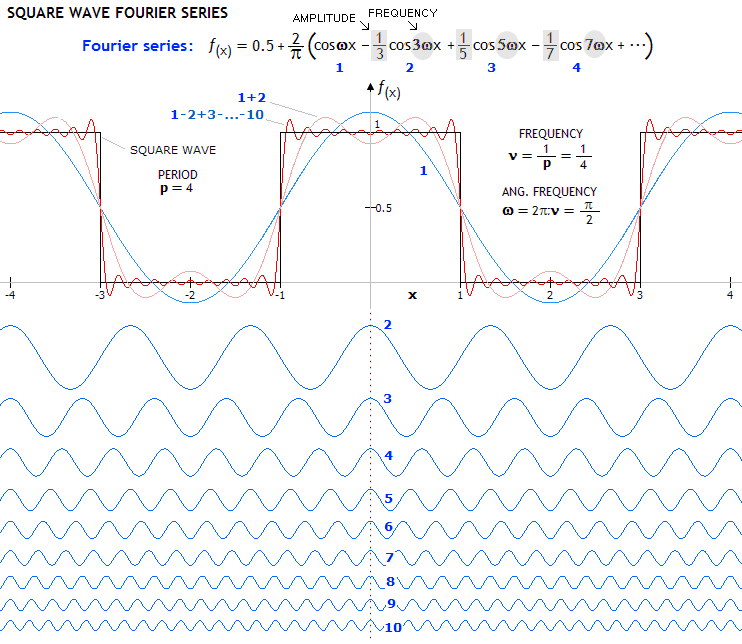Unlike the sine pattern, square wave pattern will not be reproduced as the original pattern in coherent light, even if it is of low frequency and with many orders captured. One form of distortion - ringing edge - is readily visible, and does not significantly subside in the magnitude with the increase in the number of terms (i.e. orders caught by the lens), only becomes more dense. On the other hand, incoherent light with its randomly fluctuating diffraction orders will much more evenly fill the edges with energy, forming better defined image. As the number of terms increases (since the function is even, all sine terms are zero), the sum of terms more closely approaches square wave form.
The amplitude coefficients for each frequency are the basis for the frequency spectrum associated with square wave function, i.e. they describe its Fourier transform. Unlike sinusoidal wave, having a single frequency amplitude in the positive domain (i.e. forming a single bright off-center spot in the Fourier plane), frequency spectrum for square wave distribution is enveloped within sinc function, with an infinite number of steadily decaying discrete frequencies, as this series indicates (in other words, it will form an infinite number of off-center bright points lined up in the Fourier plane, steadily diminishing in intensity the farther off they are). Each of those points signifies one diffraction order originating at the grid and spreading wide. The more orders are being caught by the lens bringing the Fourier transform of the square wave field to the focal plane, the better defined the image formed in the image plane (as illustrated in the Abbe model above).
Graph below shows the square wave frequency spectrum in its sinc function envelope. The unit frequency is for a sinusoid with the same period as the square wave, with the odd numbers on the horizontal scale corresponding to the numbers for the Fourier series terms given above (1 on the scale for 1 in the series, 3 on the scale to 2 in the series, and so on, since the frequencies are increasing with an odd multiplier). The amplitude for each harmonics is (2/
π)A, with A being the numerical constant for the term (for instance, A=1 for the first term, and its relative amplitude in the frequency spectrum is 2/π). As mentioned, all sine terms of the Fourier series for even function, hence for the transform too - falling on even frequency integers of the frequency spectrum - are zero. This is obvious here, since the basic frequency is π/2 (90 degrees), which gives zero when multiplied with any integer.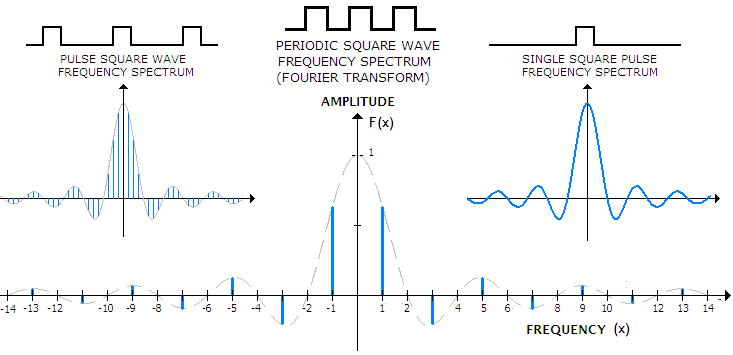As periodic wave transforms into pulse wave, with pulse separation greater than their length, relative frequency of the harmonics diminishes, with the corresponding frequency spectrum becoming more dense (top left, for the pulse wave with the period double that of the periodic square wave). When pulse separation reaches infinity, the function becomes a single square pulse, and its frequency spectrum - i.e. Fourier transform - becomes continuous sinc function (top right). Continuity of the frequency spectrum imply that the amplitude distribution in the Fourier plane is not in the form of discrete points, rather a continuous amplitude/intensity distribution, with each point of light in it signifying specific, corresponding to it harmonic frequency.
It should be noted that, formally, "Fourier transform" generally refers to the continuous spectrum function, such as a single pulse, as opposed to "discrete Fourier transform", referring to the discrete spectrum of a periodic function.
Fourier transform of a single square pulse is particularly important, because it (square pulse) is a function describing aberration-free exit pupil of an optical system with even transmission over the pupil area.
Consequently, we recognize in its Fourier transform the familiar shape of the coherent (point) spread function (CSF, which is, in effect, point amplitude-image), whose squared modulus (magnitude) is the PSF.
In turn, pupil function is the basis for calculating ATF and OTF. While the ATF integral shows that it is merely a scaled pupil function, with amplitude transfer equal to 1 for frequencies lower than 1/2λF and equal to 0 for higher frequencies, OTF is equal to the autocorrelation of its pupil function. With pupil function defined by the amplitude (electric field) and phase in the plane of exit pupil, the aberration free pupil with even transmission is a square wave function in 2-D, and a cylinder in 3-D. With pupil amplitude normalized to 1, OTF is proportional to the area of overlap of the pupil in autocorrelation (strictly, to the volume of overlap, but for a cylinder-like function there is no difference), relative to the pupil area, normalized to one. At the cutoff frequency - when the two centers are separated by λf
νC, with νC=1/λF and f the focal length (generally the image separation) - the two centers are at the separation equaling pupil diameter.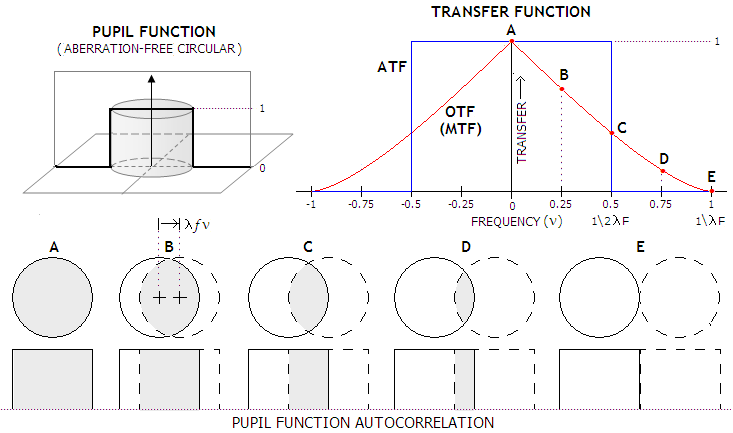Pupil function and, as expected, its autocorrelation, becomes more complicated for aberrated systems. Pupil function can be written as P(x,y)=T(x,y)ei2πW, where T is the transmission over the pupil, e is the natural logarithm base, and W is the aberration function expressing wavefront deviation in wavelengths (x, y are the coordinates in the pupil plane). The  factor ei2πW defines phase variation over the pupil in a complex exponential notation; the reason it is the standard base for expressing complex numbers is that eix function coincides with the wave cycle, i.e. describes a sinusoid that repeats with the x+2π period. These two parts of the pupil function are not directly compatible, with the transmission T directly expressing field (amplitude) in the pupil, and the phase factor ei2πW  expressing the phase in the normalized 1 to -1 range. Given in units of wavelength, W numerically corresponds to the phase angle φ in units of the 2π (radians) full phase, thus the identity ei2πW=eiφ=cosφ+isinφ effectively gives the two coordinates (it's never a sum) determining the corresponding phase angle φ in the unit circle, as illustrated below (while this phase angle could also be expressed using ordinary numbers, the complex exponential form is generally preferred for ease of calculation and versatility).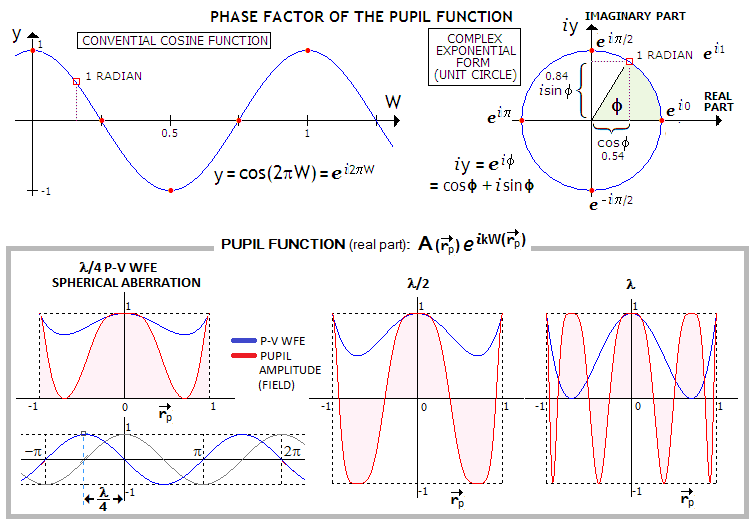This phase factor of the pupil function can be converted to the resulting amplitude at the focus point, in proportion to cos(OPD2
π/λ), which is then describing the profile of pupil function. This is illustrated (bottom) for primary spherical aberration, 1/4, 1/2 and 1 wave P-V in magnitude (since OPD for primary spherical is described by x4-x2, which for the maximum deviation for x=0.707 gives -0.25, it in effect represents 1/4 wave error, while for 1/2 and 1 wave error 2π is replaced with 4π and 8π, respectively). For the wavefront error W=0, pupil phase factor is 1, the entire pupil is in phase, hence can be represented as a cylindrical volume of the height determined by the (unit) amplitude A. With 1/4 wave of spherical aberration, all points except the center and edge are out of phase at the best focus location, with their amplitude contribution depending on the phase difference (for 1/4 wave, or π/2, difference, at the 0.707 zone, amplitude contribution from that point is 0, as illustrated below graph at left). The transfer function in incoherent light (OTF) for aberrated pupil can be also derived from the pupil autocorrelation integral, i.e. as the common volume shared by two identical pupil functions as one of them shifts to the right. The starting point, when the two coincide, is zero frequency, and the cutoff is when the two are only touching.
Therefore, a change in pupil function results in transfer function change (aberrated pupil will, of course, impair coherent amplitude transfer as well), generally impairment, and it is only logical since amplitude spread function (ASF) and its squared modulus (magnitude), the PSF, produced by the pupil are also changed (and also generally to the worse). There is no simple explanation as to why is the field (amplitude) diffracted to infinity (and brought to the image plane by lens focusing) at the exit pupil proportional to the Fourier transform of the pupil function - which is, proportional to its frequency spectrum - but the cause is ultimately in the wave nature of light. Similarly, OTF is the Fourier transform of the PSF, itself proportional to the squared ASF. Shown below are these and some other Fourier pairs. Any such pairs are merely two different forms of representing one same wave phenomenon: one in the spatial/time, the other in the frequency context.Left side shows a function describing field in the exit pupil in terms of its spatial properties, i.e. period and amplitude. At right is the corresponding Fourier transform, as the spectra of specific sinusoidal frequencies with specific amplitudes which, when summed up, produce the pupil field function. Inset with each function illustrate its 2-D intensity distribution (basically, top view of the 3-D function distribution).
For a point pulse in the pupil, Fourier transform is a constant over all frequencies, i.e. the field at infinity has constant amplitude (plane wave). Sinusoidal pupil function produces a 3-point actual pattern, but the Fourier transform does not include the brightest, central point (zero-order, i.e. zero-frequency term, called DC term, expressing average brightness for all points; for the sinusoid shown, centered around horizontal axis, the average of positive and negative amplitudes is zero, but the energy is, of course, always positive and it appears in the actual image) only the points generated by the two first-order terms. The square wave amplitude pattern in the spatial domain transforms into a line of discrete points diminishing in brightness toward higher frequencies, with their amplitudes contained in a sinc function envelope (these points of light, if sufficiently magnified, will show the familiar point-image diffraction pattern). With either sinusoidal or square wave, the increase in spatial period results in shrinking of its frequency spectrum (i.e. shift toward lower frequencies) i.e. narrowing in the separation between bright point in the actual image of the transform. The square pulse function - which is the central cross section of the aberration-free pupil function - has as its frequency transform sinc function (shown is the relation between the spatial period a and frequency for square aperture; for circular aperture sinc function hits first zero at 1.22/a). Fourier transform of a triangular pulse is sinc
2, i.e. the square aperture PSF (either shape is slightly different for circular aperture). The corresponding intensity is proportional to this transform squared, i.e. to sinc4, which implies significant further weakening of the ring structure, i.e. profile closer to Gaussian.  Gaussian pulse transforms into another Gaussian. Similarly to the discrete Fourier transforms for periodic functions (sinusoid, square wave), increase in the pulse spatial period (i.e. in the pupil diameter) results in shrinking of its frequency spectrum, i.e. shrinking of the corresponding intensit
y distribution of the field diffracted to infinity (or brought to a finite distance by a focusing lens), and the other way around.

All this can be summed up as shown below. With either coherent or incoherent light, it starts with pupil function (in this case for circular aberration-free aperture), whose Fourier transform - the amplitude spread function (ASF) - is the system's impulse response for coherent light. Pupil function along any single diameter is the square pulse, and for the full circle it is a cylinder, with unit amplitude over pupil area, and zero outside of it (the pulse and cylinder are referred to as 1-D and 2-D pupil function, respectively, not because they themselves are 1-D and 2-D, but because they originate from 1-D and 2-D pupil elements). For incoherent light, system impulse response is the squared modulus (magnitude) of ASF, the point spread function (PSF). The image in real space is formed by convolution of the Gaussian image and system impulse response. In the frequency domain, both object and image have their frequency spectrum - as the Fourier transform of their respective intensity functions - with the image spectrum being a product of object frequency spectrum and the transfer function. The transfer function itself is merely the scaled version of pupil function for coherent light, and pupil autocorrelation for incoherent light.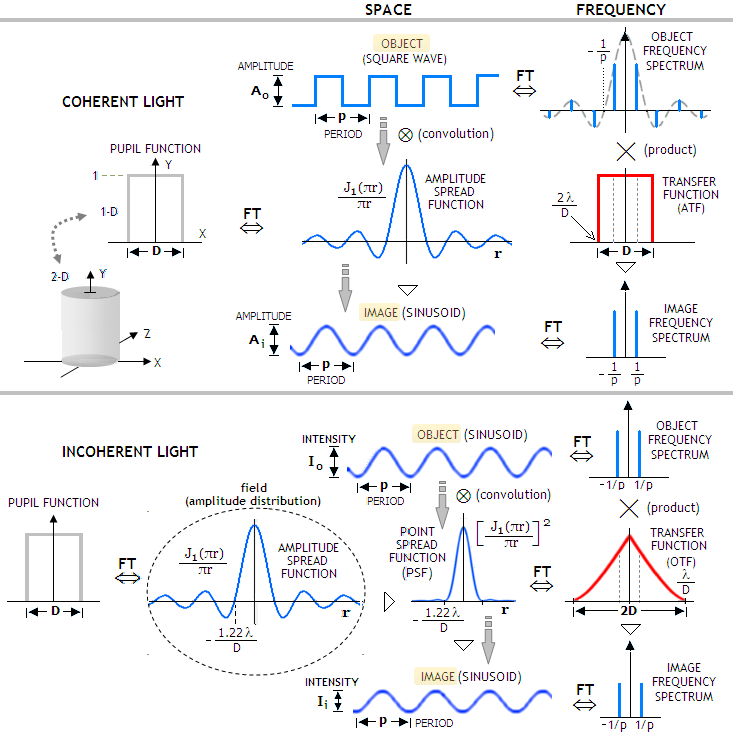In effect, the system impulse response acts as a smoothing spatial filter, softening edges, reducing amplitude, and suppressing high frequency distributions. In the frequency domain, system's transfer function acts as a low pass frequency filter. It is illustrated with coherent transfer function (top right) reducing frequency spectrum of a periodic square wave to a single frequency, whose inverse Fourier transform is the sinusoidal amplitude distribution of the image.
Note that image formation in coherent light is in the context of amplitude, since amplitudes of coherent waves add up directly as the convolution of the system's ASF and Gaussian amplitude image, and it is the resulting complex amplitude squared that determines the intensity. With incoherent light, the phase of individual waves is not correlated, and they add up their intensities directly, as the convolution of the system PSF and Gaussian image. Only for point source there is no difference in image formation in coherent vs. incoherent light (as long as it concerns the relative, not absolute intensity distribution). The PSF is the Fourier transform of the OTF, which means that it represents the amplitude distribution resulting from OTF-like pulse. Its intensity is the PSF squared, i.e. the 4th power of the ASF, with the ring structure nearly vanishing (as it is to expect, with the OTF-like pulse having in general similar shape to the Gaussian pulse (i.e. central maxima steadily diminishing toward zero going away from it), whose Fourier transform is another Gaussian.

As mentioned before, the coherent transfer function (ATF) formalism expresses only the transfer of wave amplitude (i.e. field), and does not supply information about the image itself, as OTF does. Thus, despite the ATF indicating that the coherent light imagery is perfect for the range of frequencies lower than 1/2λF (inverse linear frequency, with linear frequency given as a product of angular frequency in radians with the focal length), incoherent imaging is generally preferable over the entire range of frequencies.

And, as illustration shows, the imaging setup is relevant for microscopy, not astronomical observations. In the optical telescope, image plane and focal plane practically coincide, and the Fourier transform would have been taking place in the image, as coherent spread functions (CSF) created by light emitted from each object point and diffracted at the telescope entrance pupil, interfering in the image at the amplitude level. But even with near monochromatic (i.e. temporally coherent) light, spatial coherence in the visual astronomy is only possible over very small, near point-like patches. Extending size of the emitting area rapidly makes light spatially incoherent. Thus, ATF is even less relevant for astronomical imaging, where coherent light may be encountered only on a very small angular scale, and image forming is subject to CTF amplitude interference.

6.6.1. MTF - aberration compounding, CCD, limitations   ▐    7. OBSTRUCTION EFFECTS